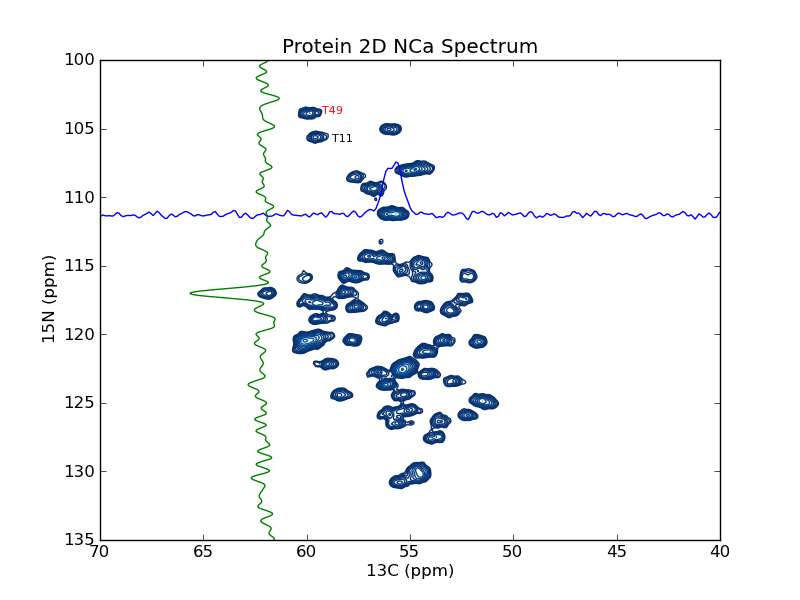# plotting example: plot_2d_spectrum

This example shows how to use nmrglue and matplotlib to create figures for examining data or publication. In this example a contour plot of the spectrum from a 2D NMRPipe file is created. Slices are added in the 15N and 13C dimension as well as sample peak labels. plotting example: plot_2d_spectrum_pts is similar to this example but plotted on a point scale.

```#! /usr/bin/env python
# Create a contour plot of a 2D NMRPipe spectrum

import nmrglue as ng
import numpy as np
import matplotlib.pyplot as plt
import matplotlib.cm

# plot parameters
cmap = matplotlib.cm.Blues_r    # contour map (colors to use for contours)
contour_start = 30000           # contour level start value
contour_num = 20                # number of contour levels
contour_factor = 1.20          # scaling factor between contour levels

# calculate contour levels
cl = contour_start * contour_factor ** np.arange(contour_num)

# read in the data from a NMRPipe file

# make ppm scales
uc_13c = ng.pipe.make_uc(dic, data, dim=1)
ppm_13c = uc_13c.ppm_scale()
ppm_13c_0, ppm_13c_1 = uc_13c.ppm_limits()
uc_15n = ng.pipe.make_uc(dic, data, dim=0)
ppm_15n = uc_15n.ppm_scale()
ppm_15n_0, ppm_15n_1 = uc_15n.ppm_limits()

# create the figure
fig = plt.figure()

# plot the contours
ax.contour(data, cl, cmap=cmap,
extent=(ppm_13c_0, ppm_13c_1, ppm_15n_0, ppm_15n_1))

ax.text(59.25, 104.0, "T49", size=8, color='r')
ax.text(58.75, 106, "T11", size=8, color='k')

# plot slices in each direction
xslice = data[uc_15n("111.27 ppm"), :]
ax.plot(ppm_13c, -xslice / 4.e4 + 111.27)
yslice = data[:, uc_13c("62.0 ppm")]
ax.plot(yslice / 2.e4 + 62.0, ppm_15n)

# decorate the axes
ax.set_ylabel("15N (ppm)")
ax.set_xlabel("13C (ppm)")
ax.set_title("Protein 2D NCa Spectrum")
ax.set_xlim(70, 40)
ax.set_ylim(135, 100)

# save the figure
fig.savefig("spectrum.png") # this can be .pdf, .ps, etc
```

Result: